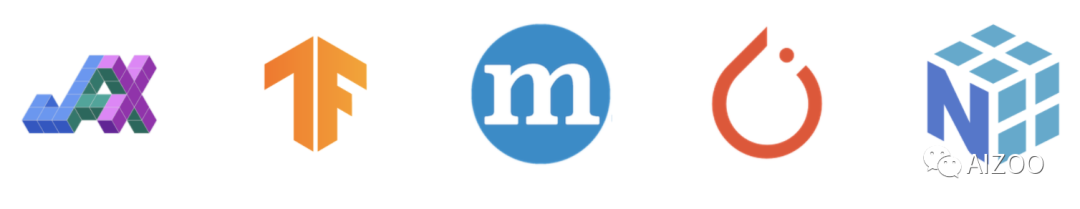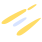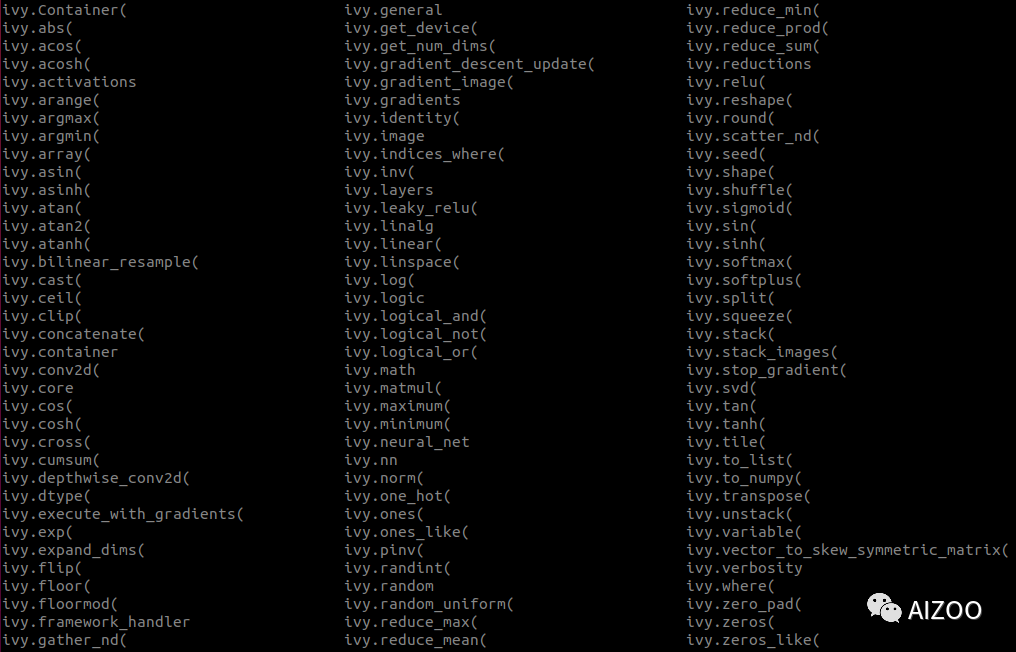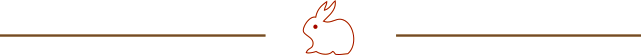# Ivy 开源，深度学习大一统时代到来？Ivy is a unified machine learning framework which maximizes the portability of machine learning codebases. Ivy wraps the functional APIs of existing frameworks. Framework-agnostic functions, libraries and layers can then be written using Ivy, with simultaneous support for all frameworks. Ivy currently supports Jax, TensorFlow, PyTorch, MXNet and Numpy.Ivy 是一个统一的机器学习框架，可最大限度地提高机器学习代码库的可移植性。Ivy 封装了现有框架的功能 API。然后可以使用 Ivy 编写与框架无关的函数、库和层，它可以同时支持所有框架。Ivy 目前支持 Jax、TensorFlow、PyTorch、MXNet 和 Numpy。

# 快速入门

``````import ivy

class MyModel(ivy.Module):
def __init__(self):
self.linear0 = ivy.Linear(3, 64)
self.linear1 = ivy.Linear(64, 1)
ivy.Module.__init__(self)

def _forward(self, x):
x = ivy.relu(self.linear0(x))
return ivy.sigmoid(self.linear1(x))

ivy.set_framework('torch')  # change to any framework!

model = MyModel()
x_in = ivy.array([1., 2., 3.])
target = ivy.array([0.])

def loss_fn(v):
out = model(x_in, v=v)
return ivy.reduce_mean((out - target)**2)

for step in range(100):
print('step {} loss {}'.format(step, ivy.to_numpy(loss).item()))

print('Finished training!')
``````

## 框架无关的函数

Ivy 的函数可以接受所有框架的 tensor，如下面的例子：

``````import jax.numpy as jnp
import tensorflow as tf
import numpy as np
import mxnet as mx
import torch

import ivy

jax_concatted = ivy.concatenate((jnp.ones((1,)), jnp.ones((1,))), -1)
tf_concatted = ivy.concatenate((tf.ones((1,)), tf.ones((1,))), -1)
np_concatted = ivy.concatenate((np.ones((1,)), np.ones((1,))), -1)
mx_concatted = ivy.concatenate((mx.nd.ones((1,)), mx.nd.ones((1,))), -1)
torch_concatted = ivy.concatenate((torch.ones((1,)), torch.ones((1,))), -1)
``````# 统一所有框架的目的何在？Ivy 官方发布的力学、3D 视觉、机器人和可微分环境的代码库都是用纯 Ivy 编写的。这些库为任何机器学习框架的用户提供了各种应用功能的完全可区分的实现，随时可以集成到用户的代码中。

Ivy 的另一个好处是灵活性。通过保持 Ivy 抽象的轻量级，以及齐全的功能，这使您可以完全控制您的代码。下面的示意图强调您可以选择在任何抽象级别进行开发。Original: https://blog.csdn.net/u010900574/article/details/122803632
Author: u010900574
Title: Ivy 开源，深度学习大一统时代到来？